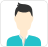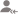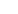truePost a Lesson

All

All

Lessons

Discussion

Lesson Posted on 02/04/2018 Tuition Tuition/Engineering Diploma Tuition/Applied Math Exam Coaching/CSIR NET +2 Matrices Tuition/BSc Tuition/BSc Mathematics lessEduDais provides Home Tuition, Group Tuition, Coaching classes and Online Tuition to school students,...

Rank of a matrix is a positive number that tells us how many rows are independent in that matrix, i.e., how many rows are not a linear combination of other rows or not a multiple of other rows. It helps us to solve a system of linear equations and to determine whether the solution exists or not. If... read more

Rank of a matrix is a positive number that tells us how many rows are independent in that matrix, i.e., how many rows are not a linear combination of other rows or not a multiple of other rows.

It helps us to solve a system of linear equations and to determine whether the solution exists or not. If the solution exists, then it is unique or not can also be determined with the help of rank.

Rank should always be greater than or equal to 1 and less than or equal to the minimum of no. of rows and no. of columns of the matrix.

Dislike Bookmark

Answered on 23/06/2017 Matrices Tuition/Class IX-X TuitionRashmitm Rashmi

Tutor

Before starting studying, first prepare the time table of your own, and mark time till you have to complete the topic and complete it in that time only. And study upto date ,don't postpone. If you make all these then no need of tuition. You will score the best.
Dislike Bookmark

How do I prepare for 10th board?How long I should study for ICSE board exam? And should I start to take tuitions?Dedicated Teacher With Lots of Teaching Experience In Maths For All Boards

Regular study of each subjects will help you a lot. Make sure that your study time for any three subjects would be 5 hours. You must do maths two hours daily. any two subjects other than maths each 1 hr 30 min. morning time is the best time to study. at least schedule two hours in the morning. Laws,... read more
Regular study of each subjects will help you a lot. Make sure that your study time for any three subjects would be 5 hours. You must do maths two hours daily. any two subjects other than maths each 1 hr 30 min. morning time is the best time to study. at least schedule two hours in the morning. Laws, its proofs must be mugged and reproduced as given in the text. These five hours of study is apart from school timings and tuition timings. read less
Dislike Bookmark

Answered on 14/08/2017 CBSE/Class 12/Science/Mathematics Tuition/Class XI-XII Tuition (PUC) MatricesDevendra Singh

Tutor

Replace Aij with Aji... Where Aij is the element of A matrix corresponding to i'th row and j'th column.
Dislike Bookmark

Answered on 02/12/2016 CBSE/Class 12/Science/Mathematics Tuition/Class XI-XII Tuition (PUC) Matrices

What do you mean by order of matrix?Rajesh Chilla

Math Teacher

The no. of rows and columns in matrix.
Dislike Bookmark

Answered on 08/12/2016 CBSE/Class 12/Science/Mathematics Tuition/Class XI-XII Tuition (PUC) Matrices

Define column matrix and row matrix?Shreyans

Software Engineer

Column matrix : 1 or more than 1 row and only 1 column matrix called column matrix . Row matrix : 1 or more than 1 column and only 1 row matrix called row matrix . e.g it's 3*1.
Dislike Bookmark

Answered on 01/12/2016 CBSE/Class 12/Science/Mathematics Tuition/Class XI-XII Tuition (PUC) Matrices

Define square matrix?Rajesh Chilla

Math Teacher

A matrix consist of m rows and n column, where m = n.
Dislike Bookmark

Answered on 27/11/2016 CBSE/Class 12/Science/Mathematics Tuition/Class XI-XII Tuition (PUC) Matrices

What do you mean by equality of matrix?Tapas Bhattacharya

Tutor

If each matrix has:- (1) the same number of rows, (2) the same number of columns and (3) corresponding elements within each matrix are equal then the two matrices are said to be equal.
Dislike Bookmark

Answered on 03/12/2016 CBSE/Class 12/Science/Mathematics Tuition/Class XI-XII Tuition (PUC) Matrices

If A = [9 0 8], B = [6 8 9] then find AB, BA. Show that AB = BA?Sarvajeet Kumar

An Experienced Trainer

AB=BA=O Where O=Zero matrix.
Dislike Bookmark

Answered on 08/12/2016 CBSE/Class 12/Science/Mathematics Tuition/Class XI-XII Tuition (PUC) Matrices

If A = [6 8 4], B = [0 5 8], C = [5 23 31]. Then find A(BC), (AB)C?Shreyans

Software Engineer

First of all it's not possible to multiply matrix as per rule. If I want to multiply tow matrix first matrix column and second matrix row should be same.
Dislike Bookmark

UrbanPro.com helps you to connect with the best in India. Post Your Requirement today and get connected.

Overview

Questions 27

Total Shares44 Followers
x

X

### Find Tutors, Trainers & Institutes near you

Post requirement and connect with the tutors in your locality

• Post a learning requirement
• Get customized responses
• Compare and select the best### Want to learn something New?

Find best tutors, trainers & institutes near you on UrbanProUrbanPro.com is India's largest network of most trusted tutors and institutes. Over 25 lakh students rely on UrbanPro.com, to fulfill their learning requirements across 1,000+ categories. Using UrbanPro.com, parents, and students can compare multiple Tutors and Institutes and choose the one that best suits their requirements. More than 6.5 lakh verified Tutors and Institutes are helping millions of students every day and growing their tutoring business on UrbanPro.com. Whether you are looking for a tutor to learn mathematics, a German language trainer to brush up your German language skills or an institute to upgrade your IT skills, we have got the best selection of Tutors and Training Institutes for you. Read more